Publicité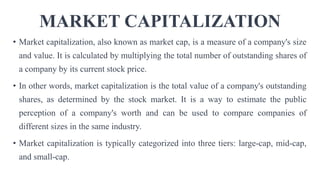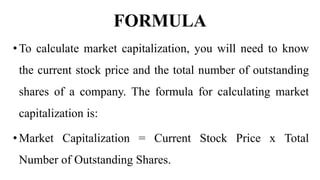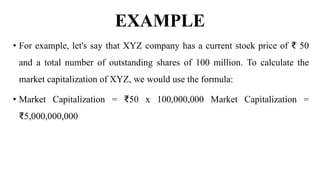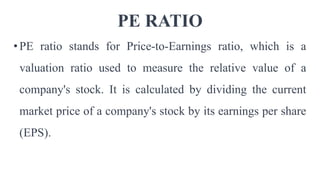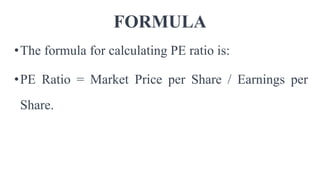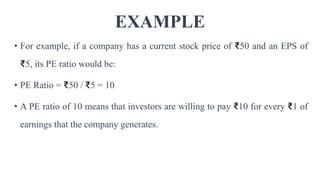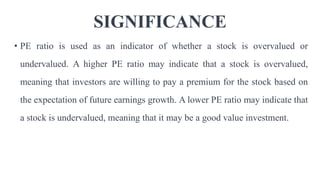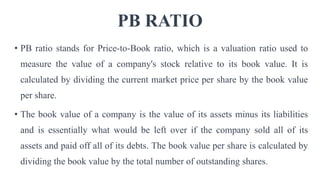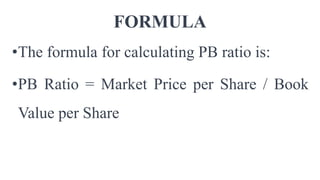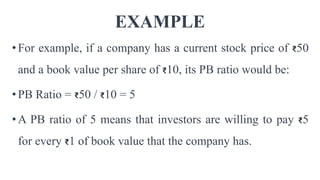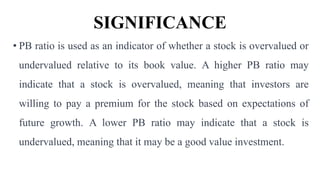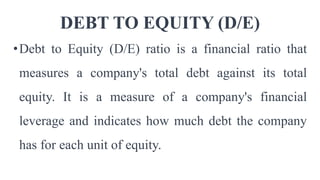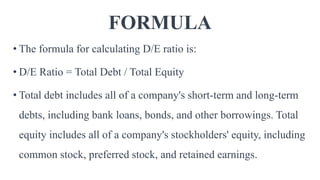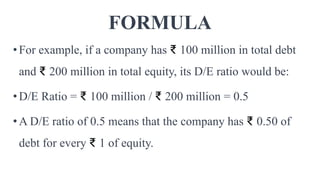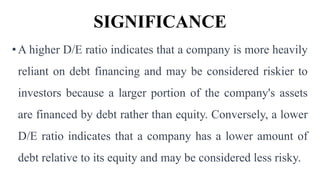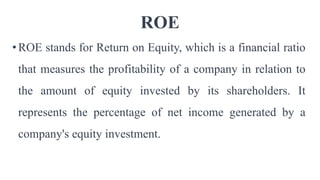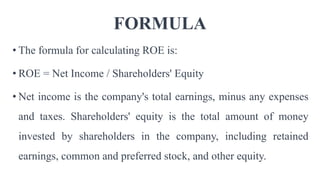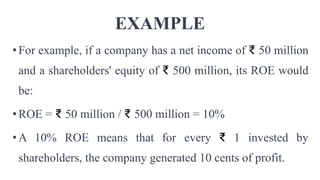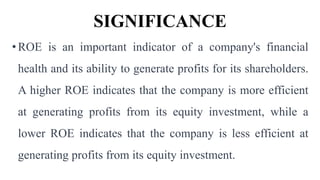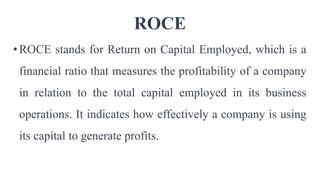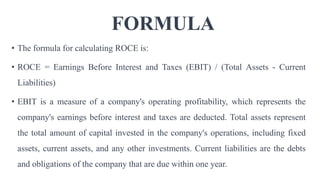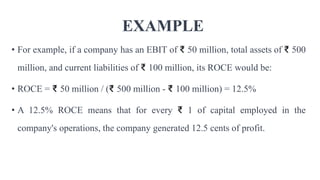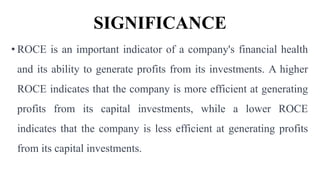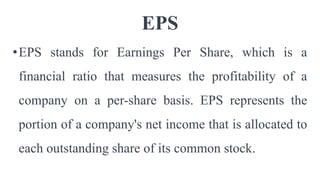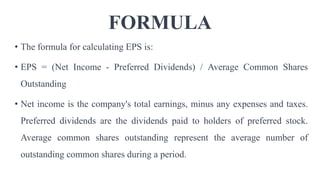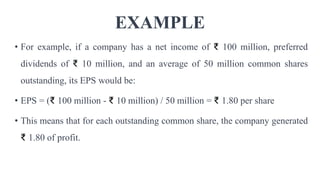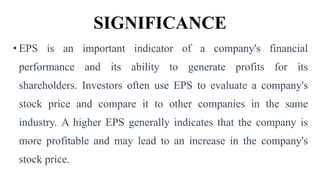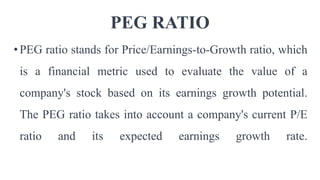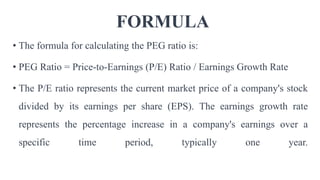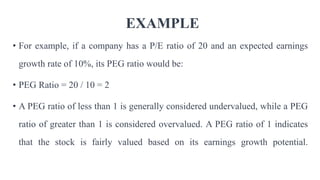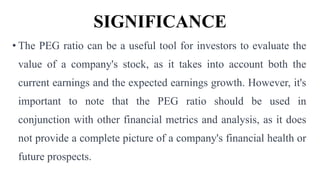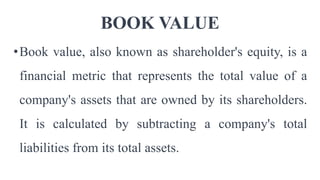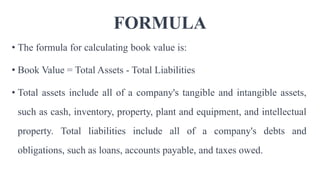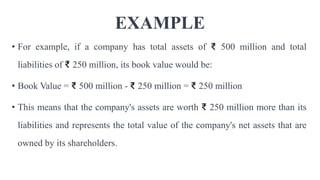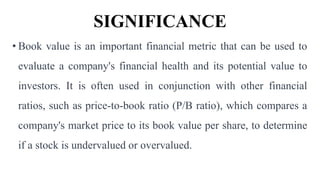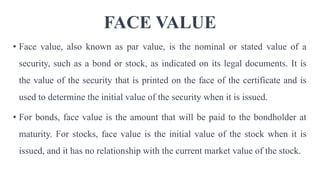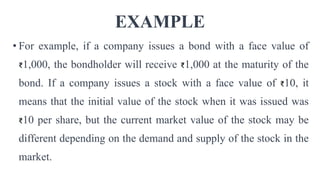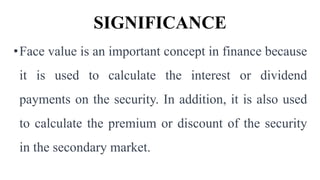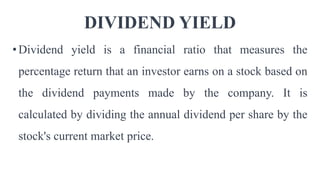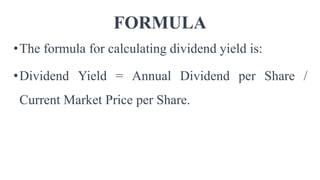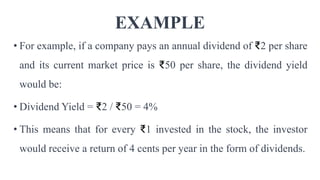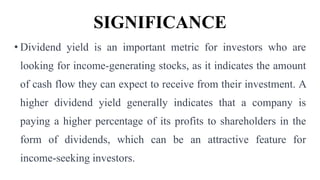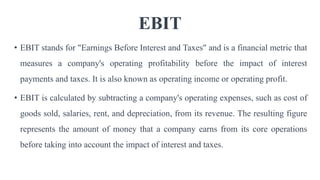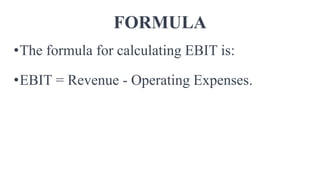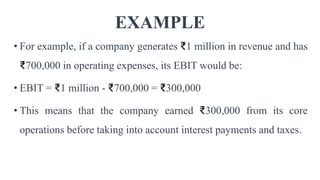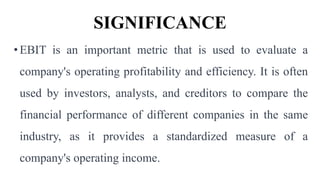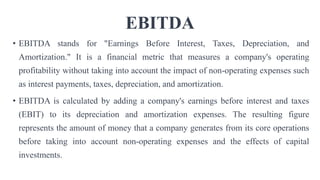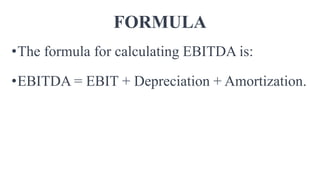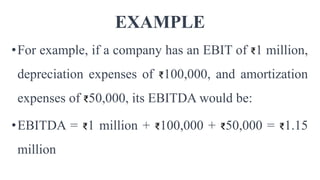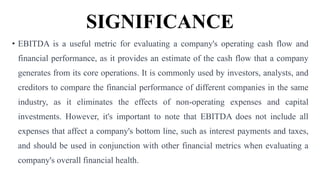1 sur 52
Publicité

### INTRODUCTION TO FINANCIAL METRICS FOR STOCK MARKET ANALYSIS.pptx

1. INTRODUCTION TO FINANCIAL METRICS FOR STOCK MARKET ANALYSIS BY MONOJIT GOPE RESEARCH SCHOLAR
2. MARKET CAPITALIZATION • Market capitalization, also known as market cap, is a measure of a company's size and value. It is calculated by multiplying the total number of outstanding shares of a company by its current stock price. • In other words, market capitalization is the total value of a company's outstanding shares, as determined by the stock market. It is a way to estimate the public perception of a company's worth and can be used to compare companies of different sizes in the same industry. • Market capitalization is typically categorized into three tiers: large-cap, mid-cap, and small-cap.
3. FORMULA •To calculate market capitalization, you will need to know the current stock price and the total number of outstanding shares of a company. The formula for calculating market capitalization is: •Market Capitalization = Current Stock Price x Total Number of Outstanding Shares.
4. EXAMPLE • For example, let's say that XYZ company has a current stock price of ₹ 50 and a total number of outstanding shares of 100 million. To calculate the market capitalization of XYZ, we would use the formula: • Market Capitalization = ₹50 x 100,000,000 Market Capitalization = ₹5,000,000,000
5. PE RATIO •PE ratio stands for Price-to-Earnings ratio, which is a valuation ratio used to measure the relative value of a company's stock. It is calculated by dividing the current market price of a company's stock by its earnings per share (EPS).
6. FORMULA •The formula for calculating PE ratio is: •PE Ratio = Market Price per Share / Earnings per Share.
7. EXAMPLE • For example, if a company has a current stock price of ₹50 and an EPS of ₹5, its PE ratio would be: • PE Ratio = ₹50 / ₹5 = 10 • A PE ratio of 10 means that investors are willing to pay ₹10 for every ₹1 of earnings that the company generates.
8. SIGNIFICANCE • PE ratio is used as an indicator of whether a stock is overvalued or undervalued. A higher PE ratio may indicate that a stock is overvalued, meaning that investors are willing to pay a premium for the stock based on the expectation of future earnings growth. A lower PE ratio may indicate that a stock is undervalued, meaning that it may be a good value investment.
9. PB RATIO • PB ratio stands for Price-to-Book ratio, which is a valuation ratio used to measure the value of a company's stock relative to its book value. It is calculated by dividing the current market price per share by the book value per share. • The book value of a company is the value of its assets minus its liabilities and is essentially what would be left over if the company sold all of its assets and paid off all of its debts. The book value per share is calculated by dividing the book value by the total number of outstanding shares.
10. FORMULA •The formula for calculating PB ratio is: •PB Ratio = Market Price per Share / Book Value per Share
11. EXAMPLE •For example, if a company has a current stock price of ₹50 and a book value per share of ₹10, its PB ratio would be: •PB Ratio = ₹50 / ₹10 = 5 •A PB ratio of 5 means that investors are willing to pay ₹5 for every ₹1 of book value that the company has.
12. SIGNIFICANCE • PB ratio is used as an indicator of whether a stock is overvalued or undervalued relative to its book value. A higher PB ratio may indicate that a stock is overvalued, meaning that investors are willing to pay a premium for the stock based on expectations of future growth. A lower PB ratio may indicate that a stock is undervalued, meaning that it may be a good value investment.
13. DEBT TO EQUITY (D/E) •Debt to Equity (D/E) ratio is a financial ratio that measures a company's total debt against its total equity. It is a measure of a company's financial leverage and indicates how much debt the company has for each unit of equity.
14. FORMULA • The formula for calculating D/E ratio is: • D/E Ratio = Total Debt / Total Equity • Total debt includes all of a company's short-term and long-term debts, including bank loans, bonds, and other borrowings. Total equity includes all of a company's stockholders' equity, including common stock, preferred stock, and retained earnings.
15. FORMULA •For example, if a company has ₹ 100 million in total debt and ₹ 200 million in total equity, its D/E ratio would be: •D/E Ratio = ₹ 100 million / ₹ 200 million = 0.5 •A D/E ratio of 0.5 means that the company has ₹ 0.50 of debt for every ₹ 1 of equity.
16. SIGNIFICANCE •A higher D/E ratio indicates that a company is more heavily reliant on debt financing and may be considered riskier to investors because a larger portion of the company's assets are financed by debt rather than equity. Conversely, a lower D/E ratio indicates that a company has a lower amount of debt relative to its equity and may be considered less risky.
17. ROE •ROE stands for Return on Equity, which is a financial ratio that measures the profitability of a company in relation to the amount of equity invested by its shareholders. It represents the percentage of net income generated by a company's equity investment.
18. FORMULA • The formula for calculating ROE is: • ROE = Net Income / Shareholders' Equity • Net income is the company's total earnings, minus any expenses and taxes. Shareholders' equity is the total amount of money invested by shareholders in the company, including retained earnings, common and preferred stock, and other equity.
19. EXAMPLE •For example, if a company has a net income of ₹ 50 million and a shareholders' equity of ₹ 500 million, its ROE would be: •ROE = ₹ 50 million / ₹ 500 million = 10% •A 10% ROE means that for every ₹ 1 invested by shareholders, the company generated 10 cents of profit.
20. SIGNIFICANCE •ROE is an important indicator of a company's financial health and its ability to generate profits for its shareholders. A higher ROE indicates that the company is more efficient at generating profits from its equity investment, while a lower ROE indicates that the company is less efficient at generating profits from its equity investment.
21. ROCE •ROCE stands for Return on Capital Employed, which is a financial ratio that measures the profitability of a company in relation to the total capital employed in its business operations. It indicates how effectively a company is using its capital to generate profits.
22. FORMULA • The formula for calculating ROCE is: • ROCE = Earnings Before Interest and Taxes (EBIT) / (Total Assets - Current Liabilities) • EBIT is a measure of a company's operating profitability, which represents the company's earnings before interest and taxes are deducted. Total assets represent the total amount of capital invested in the company's operations, including fixed assets, current assets, and any other investments. Current liabilities are the debts and obligations of the company that are due within one year.
23. EXAMPLE • For example, if a company has an EBIT of ₹ 50 million, total assets of ₹ 500 million, and current liabilities of ₹ 100 million, its ROCE would be: • ROCE = ₹ 50 million / (₹ 500 million - ₹ 100 million) = 12.5% • A 12.5% ROCE means that for every ₹ 1 of capital employed in the company's operations, the company generated 12.5 cents of profit.
24. SIGNIFICANCE • ROCE is an important indicator of a company's financial health and its ability to generate profits from its investments. A higher ROCE indicates that the company is more efficient at generating profits from its capital investments, while a lower ROCE indicates that the company is less efficient at generating profits from its capital investments.
25. EPS •EPS stands for Earnings Per Share, which is a financial ratio that measures the profitability of a company on a per-share basis. EPS represents the portion of a company's net income that is allocated to each outstanding share of its common stock.
26. FORMULA • The formula for calculating EPS is: • EPS = (Net Income - Preferred Dividends) / Average Common Shares Outstanding • Net income is the company's total earnings, minus any expenses and taxes. Preferred dividends are the dividends paid to holders of preferred stock. Average common shares outstanding represent the average number of outstanding common shares during a period.
27. EXAMPLE • For example, if a company has a net income of ₹ 100 million, preferred dividends of ₹ 10 million, and an average of 50 million common shares outstanding, its EPS would be: • EPS = (₹ 100 million - ₹ 10 million) / 50 million = ₹ 1.80 per share • This means that for each outstanding common share, the company generated ₹ 1.80 of profit.
28. SIGNIFICANCE • EPS is an important indicator of a company's financial performance and its ability to generate profits for its shareholders. Investors often use EPS to evaluate a company's stock price and compare it to other companies in the same industry. A higher EPS generally indicates that the company is more profitable and may lead to an increase in the company's stock price.
29. PEG RATIO • PEG ratio stands for Price/Earnings-to-Growth ratio, which is a financial metric used to evaluate the value of a company's stock based on its earnings growth potential. The PEG ratio takes into account a company's current P/E ratio and its expected earnings growth rate.
30. FORMULA • The formula for calculating the PEG ratio is: • PEG Ratio = Price-to-Earnings (P/E) Ratio / Earnings Growth Rate • The P/E ratio represents the current market price of a company's stock divided by its earnings per share (EPS). The earnings growth rate represents the percentage increase in a company's earnings over a specific time period, typically one year.
31. EXAMPLE • For example, if a company has a P/E ratio of 20 and an expected earnings growth rate of 10%, its PEG ratio would be: • PEG Ratio = 20 / 10 = 2 • A PEG ratio of less than 1 is generally considered undervalued, while a PEG ratio of greater than 1 is considered overvalued. A PEG ratio of 1 indicates that the stock is fairly valued based on its earnings growth potential.
32. SIGNIFICANCE • The PEG ratio can be a useful tool for investors to evaluate the value of a company's stock, as it takes into account both the current earnings and the expected earnings growth. However, it's important to note that the PEG ratio should be used in conjunction with other financial metrics and analysis, as it does not provide a complete picture of a company's financial health or future prospects.
33. BOOK VALUE •Book value, also known as shareholder's equity, is a financial metric that represents the total value of a company's assets that are owned by its shareholders. It is calculated by subtracting a company's total liabilities from its total assets.
34. FORMULA • The formula for calculating book value is: • Book Value = Total Assets - Total Liabilities • Total assets include all of a company's tangible and intangible assets, such as cash, inventory, property, plant and equipment, and intellectual property. Total liabilities include all of a company's debts and obligations, such as loans, accounts payable, and taxes owed.
35. EXAMPLE • For example, if a company has total assets of ₹ 500 million and total liabilities of ₹ 250 million, its book value would be: • Book Value = ₹ 500 million - ₹ 250 million = ₹ 250 million • This means that the company's assets are worth ₹ 250 million more than its liabilities and represents the total value of the company's net assets that are owned by its shareholders.
36. SIGNIFICANCE • Book value is an important financial metric that can be used to evaluate a company's financial health and its potential value to investors. It is often used in conjunction with other financial ratios, such as price-to-book ratio (P/B ratio), which compares a company's market price to its book value per share, to determine if a stock is undervalued or overvalued.
37. FACE VALUE • Face value, also known as par value, is the nominal or stated value of a security, such as a bond or stock, as indicated on its legal documents. It is the value of the security that is printed on the face of the certificate and is used to determine the initial value of the security when it is issued. • For bonds, face value is the amount that will be paid to the bondholder at maturity. For stocks, face value is the initial value of the stock when it is issued, and it has no relationship with the current market value of the stock.
38. EXAMPLE • For example, if a company issues a bond with a face value of ₹1,000, the bondholder will receive ₹1,000 at the maturity of the bond. If a company issues a stock with a face value of ₹10, it means that the initial value of the stock when it was issued was ₹10 per share, but the current market value of the stock may be different depending on the demand and supply of the stock in the market.
39. SIGNIFICANCE •Face value is an important concept in finance because it is used to calculate the interest or dividend payments on the security. In addition, it is also used to calculate the premium or discount of the security in the secondary market.
40. DIVIDEND YIELD •Dividend yield is a financial ratio that measures the percentage return that an investor earns on a stock based on the dividend payments made by the company. It is calculated by dividing the annual dividend per share by the stock's current market price.
41. FORMULA •The formula for calculating dividend yield is: •Dividend Yield = Annual Dividend per Share / Current Market Price per Share.
42. EXAMPLE • For example, if a company pays an annual dividend of ₹2 per share and its current market price is ₹50 per share, the dividend yield would be: • Dividend Yield = ₹2 / ₹50 = 4% • This means that for every ₹1 invested in the stock, the investor would receive a return of 4 cents per year in the form of dividends.
43. SIGNIFICANCE • Dividend yield is an important metric for investors who are looking for income-generating stocks, as it indicates the amount of cash flow they can expect to receive from their investment. A higher dividend yield generally indicates that a company is paying a higher percentage of its profits to shareholders in the form of dividends, which can be an attractive feature for income-seeking investors.
44. EBIT • EBIT stands for "Earnings Before Interest and Taxes" and is a financial metric that measures a company's operating profitability before the impact of interest payments and taxes. It is also known as operating income or operating profit. • EBIT is calculated by subtracting a company's operating expenses, such as cost of goods sold, salaries, rent, and depreciation, from its revenue. The resulting figure represents the amount of money that a company earns from its core operations before taking into account the impact of interest and taxes.
45. FORMULA •The formula for calculating EBIT is: •EBIT = Revenue - Operating Expenses.
46. EXAMPLE • For example, if a company generates ₹1 million in revenue and has ₹700,000 in operating expenses, its EBIT would be: • EBIT = ₹1 million - ₹700,000 = ₹300,000 • This means that the company earned ₹300,000 from its core operations before taking into account interest payments and taxes.
47. SIGNIFICANCE • EBIT is an important metric that is used to evaluate a company's operating profitability and efficiency. It is often used by investors, analysts, and creditors to compare the financial performance of different companies in the same industry, as it provides a standardized measure of a company's operating income.
48. EBITDA • EBITDA stands for "Earnings Before Interest, Taxes, Depreciation, and Amortization." It is a financial metric that measures a company's operating profitability without taking into account the impact of non-operating expenses such as interest payments, taxes, depreciation, and amortization. • EBITDA is calculated by adding a company's earnings before interest and taxes (EBIT) to its depreciation and amortization expenses. The resulting figure represents the amount of money that a company generates from its core operations before taking into account non-operating expenses and the effects of capital investments.
49. FORMULA •The formula for calculating EBITDA is: •EBITDA = EBIT + Depreciation + Amortization.
50. EXAMPLE •For example, if a company has an EBIT of ₹1 million, depreciation expenses of ₹100,000, and amortization expenses of ₹50,000, its EBITDA would be: •EBITDA = ₹1 million + ₹100,000 + ₹50,000 = ₹1.15 million
51. SIGNIFICANCE • EBITDA is a useful metric for evaluating a company's operating cash flow and financial performance, as it provides an estimate of the cash flow that a company generates from its core operations. It is commonly used by investors, analysts, and creditors to compare the financial performance of different companies in the same industry, as it eliminates the effects of non-operating expenses and capital investments. However, it's important to note that EBITDA does not include all expenses that affect a company's bottom line, such as interest payments and taxes, and should be used in conjunction with other financial metrics when evaluating a company's overall financial health.
52. THANK YOU
Publicité